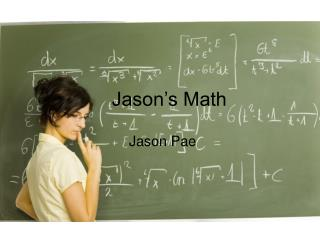DownloadDownload PresentationJason’s Math

# Jason’s Math

Download Presentation## Jason’s Math

- - - - - - - - - - - - - - - - - - - - - - - - - - - E N D - - - - - - - - - - - - - - - - - - - - - - - - - - -
##### Presentation Transcript

1. Jason’s Math Jason Pae

2. Area

3. Area

4. The area of the auditorium.

5. This is the carpet/rug. Formula 13.6m x 10.7m 145.52. 13.6m 10.7m The area is 145.52

6. Perimeter

7. Perimeter: The length around the edge of a shape

8. This is a percentage. A percentage is a number over 100. The slanted line in% is a one and the two circles next to the slanted line. So % stands for 100. 100 %

9. These are tessellations. tessellations are a pattern that doesn’t overlap.

10. These are examples of tessellations.

11. But these are not tessellations.

12. Math Problems

13. The ball is dropped from a 128 feet building. Each time it bounces it bounces half-way up than the last time. How far will the ball bounce up when it bounces on the fourth time? Here is the answer.

14. 128 ft. 64 32 16 8

15. Frog Problem: A frog fell into a 10- foot well. He has to climb out because he cannot hop out. Every day, he climbs 3 feet and every night, he slides back 2 feet. At this rate, how many days will it take poor Froggie to climb out?

16. Day 2 Day 1 Day 3 10 9 8 7 6 5 4 3 2 1 0

17. Factors

18. Factors are numbers divisible by a number. Ex. The factors of 27 are 9,3 and 1,27. Ex. The factors of 21 are 7,3 and 1,21.

19. Prime and Composite Numbers

20. Prime numbers are numbers that are only divisible by the number itself and 1. These are also prime numbers: 2, 3, 5, 7, 11, 13, 17, 19, 23, 26 and so on. 2,1 and 3,1 and 5,1 and 7,1 and 11,1 and 13,1 17,1 19, 23, 26 and so on. Factors :

21. Composite numbers are the opposite. Like 10, 20, 30, 27, 12, 15, and so on.

22. How many blocks are in this picture?

23. There are 3,151 blocks on this picture.

24. Algebra Puzzle Solution

25. There are three of the same thing so divide 9 into 3 and that gives you 3!

26. There are 2 flowers and 1 clock. The flowers equal 3 so 11 minus 6 = 5. So the clock equals 5!

27. There are 2 flowers and 1 drum. The flowers equal 3 so 16 minus 6 equals 10. The drum equals 10!

28. Tada!

29. Patterns of 11 • 112 = 121 • 1112 = 12321 • 11112 = 1234321 • 111112 = 123454321 • 1111112 = 12345654321 • 11111112 = 1234567654321 • 111111112 = 123456787654321 • 1111111112 = 12345678987654321 • 11111111112 = 1234567900987654321

30. The riders of the jungle cat’s solution.

31. Glossary of Math

32. Dozen • 12 things of the same kind.

33. Gross • 12 boxes/packages of 12. (144)

34. Baker’s Dozen • 13 things of the same kind.

35. Fortnight • 14 days.

36. Score • 20 things of the same kind.

37. Millennium • 1,000 years.

38. Bar Graph • Bar graph is a graph that uses bars to present numbers. 11 10 9 8 7 6 5 4 3 2 1 Fred Tom George

39. Century • A century is another way to express a hundred years A 100 years = a century! A century is the same as a hundred years!

40. Congruent • Two things that are same (Ex. Same size, same shape). like and

41. Reviewing Congruency

42. Congruency is when two or more things are the same size and shape. examples: and and

43. Combining Like Terms

44. First let’s start with an example. Example: 12z +17z = ? To find the answer just add 12 and 17. It is like this:12 soccer balls + 17 soccer balls = 29 soccer balls.

45. Real Algebra

46. Let’s start with this algebra problem! One number is ten times another. Their sum is 55. Find the numbers. First do this. 1. Y + 10Y = 55

47. 5 3 4 Pythagoras’s Theorem Right triangle A2 + B2 = C2

48. Mixed Numbers! • Mixed numbers are numbers that have both whole numbers and fractions. Here is an example. 2 Answer: 3 4

49. The Shifter Jason Pae